## GATE 2019

 Question 1

Ten friends planned to share equally the cost of buying a gift for their teacher. When two of them decided not to contribute, each of the other friends had to pay Rs 150 more. The cost of the gift was Rs. _____.

 A 12000 B 666 C 3000 D 6000
Aptitude       Verbal
Question 1 Explanation:
Total no. of friends = 10
When two people are not going to contribute then remaining friends have to pay Rs 150 more.
Two friends contribution = 150 × 8 = 1200
One friend contribution = 600
Ten friends contribution = 6000
Cost of the Gift = 6000

Out of ten members two members are not able to pay their share.
So, two members amount was paid by remaining eight members.
Then the eight members has to pay 150 more, which is 150*8= 1200( two persons share).
One person share= 1200/2=600.
Ten members share= 600*10= 6000.
 Question 2

The expenditure on the project _____ as follows; equipment Rs.20 lakhs, salaries Rs.12 lakhs, and contingency Rs.3 lakhs.

 A break down B break C breaks down D breaks
Aptitude       Verbal
Question 2 Explanation:
Breaks down means separated (or) classify the thing into smaller parts.
 Question 3

The search engine's business model _____ around the fulcrum of trust.

 A bursts B revolves C sinks D plays
Aptitude       Verbal
Question 3 Explanation:
Revolves: Treat as the most important element.
 Question 4

Two cars start at the same time from the same location and go in the same direction. The speed of the first car is 50 Km/h and the speed of the second car is 60 Km/h. The number of hours it takes for the distance between the two cars to be 20 Km is _____.

 A 2 B 3 C 1 D 6
Aptitude       Numerical
Question 4 Explanation:
Speed of the first car = 50 km/h
Speed of the second car = 60 km/h
Let no. of hours = x say
⇒ From the question we can write
(60)x - (50)x = 20
10x = 20
∴ x = 2 hrs
 Question 5

A court is to a judge as _____ is to a teacher.

 A a syllabus B a student C a punishment D a school
Aptitude       Verbal
Question 5 Explanation:
A court is to a judge as a school is to a teacher.
 Question 6

In a college, there are three student clubs. Sixty students are only in the Drama club, 80 students are only in the Dance club, 30 students are only in the Maths club, 40 students are in both Drama and Dance clubs, 12 students are in both Dance and Maths clubs, 7 students are in both Drama and Maths club, and 2 students are in all the clubs. If 75% of the students in the college are not in any of these clubs, then the total number of students in the college is _____.

 A 900 B 975 C 225 D 1000
Aptitude       Numerical
Question 6 Explanation:No. of students present in three student clubs
= 60 + 80 + 30 + 38 + 2 + 10 + 5
= 225 [i.e., 25% of the students in the college]
Total no. of students in the college = 225 × 4 = 900
 Question 7

The police arrested four criminals - P, Q, R and S. The criminals knew each other. They made the following statements:

P says "Q committed the crime."
Q says "S committed the crime."
R says "I did not do it."
S says "What Q said about me is false."

Assume only one of the arrested four committed the crime and only one of the statements made above is true. Who committed the crime?

 A P B Q C R D S
Aptitude       Verbal
Question 7 Explanation:
⇒ I true: 'Q' is Criminal
II, III, IV are false.
II is false: 'S' is not criminal.
IV is also false: 'S' committed crime.
There is conflict among I, IV now as P, S both should be criminals.
⇒ II true: 'S' is criminal.
I, III, IV are false.
IV is false. So, 'S' is not criminal.
III is false. So. 'R' is criminal.
There is conflict among II, III as S, R both should be criminal.
III is true: Criminal ≠ R.
I, II, IV are false.
I is false. So, 'Q' is also not criminal.
II is false. So, 'S' is not criminal.
IV is false. So, 'S' is not criminal.
Q, R, S are not criminals. So, 'P' is criminal.
IV is true: I, II, III are false.
II is false. So, 'S' is not criminal.
IV is true. So, 'S' is criminal.
I is false. So, 'Q' is not criminal.
III is false. So, 'R' is criminal.
There is conflict, as R, S both should be criminals.
 Question 8

"A recent High Court judgement has sought to dispel the idea of begging as a disease — which leads to its stigmatization and criminalization — and to regard it as a symptom. The underlying disease is the failure of the state to protect citizens who fall through the social security net."

Which of the following statements can be inferred from the given passage?

 A Beggars are created because of the lack of social welfare schemes B Beggars are lazy people who beg because they are unwilling to work C Begging is an offence that has to be dealt with firmly D Begging has to be banned because it adversely affects the welfare of the state
Aptitude       Verbal
Question 8 Explanation:
Beggars are created because of the lack of social welfare scheme.
The court is judged that because of failure of the state to protect citizens through social security schemes beggars are created.
 Question 9

Three of the five students allocated to a hostel put in special requests to the warden. Given the floor plan of the vacant rooms, select the allocation plan that will accommodate all their requests.

Request by X: Due to pollen allergy, I want to avoid a wing next to the garden.
Request by Y: I want to live as far from the washrooms as possible, since I am very sensitive to smell.
Request by Z: I believe in Vaastu and so want to stay in the South-west wing.

 A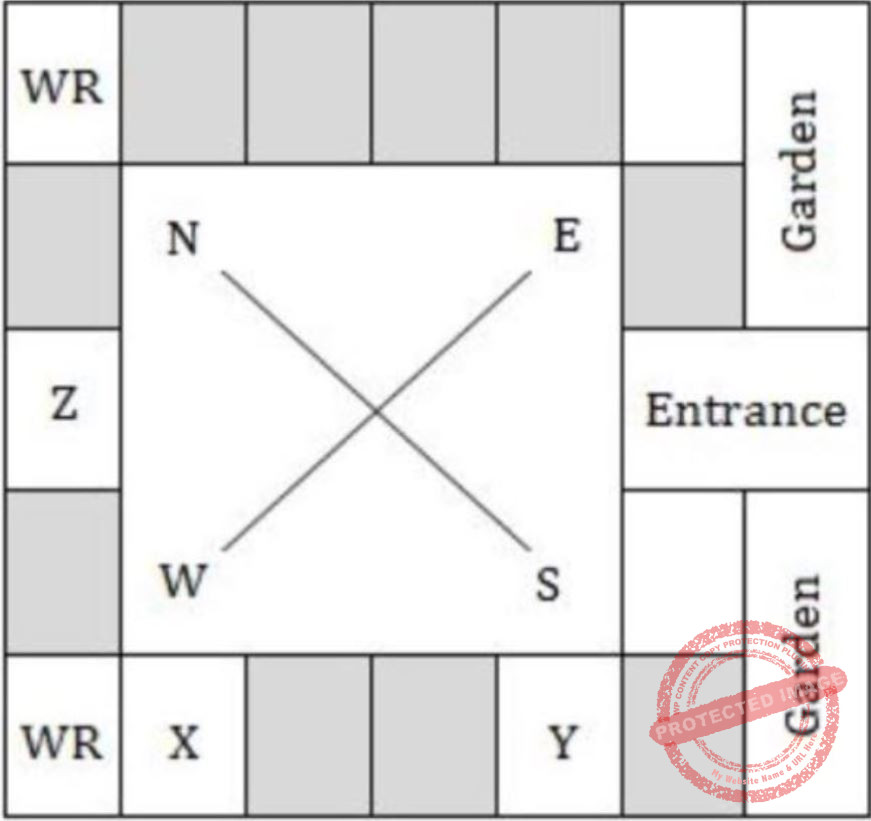BCDAptitude       Numerical
Question 9 Explanation:
Consider Option D, which satisfies all the given three statements.
 Question 10

In the given diagram, teachers are represented in the triangle, researchers in the circle and administrators in the rectngle. Out of the total number of the people, the percentage of administrators shall be in the range of _____.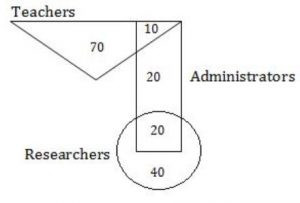A 16 to 30 B 0 to 15 C 46 to 60 D 31 to 45
Aptitude       Numerical
Question 10 Explanation: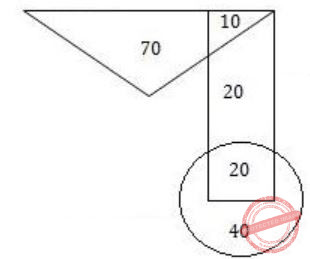From the given diagram:Total no. of people = 70 + 10 + 20 + 20 + 40 = 160
% of Administrators = 50/160 = 31.25
 Question 11

If L is a regular language over Σ = {a,b}, which one of the following languages is NOT regular?

 A Suffix (L) = {y ∈ Σ* such that xy ∈ L} B {wwR │w ∈ L} C Prefix (L) = {x ∈ Σ*│∃y ∈ Σ* such that xy ∈ L} D L ∙ LR = {xy │ x ∈ L, yR ∈ L}
Theory-of-Computation       Regular-Language
Question 11 Explanation:
wwR cannot be recognized without using stack, so it cannot be regular.
 Question 12

The chip select logic for a certain DRAM chip in a memory system design is shown below. Assume that the memory system has 16 address lines denoted by A15 to A0. What is the range of addresses (in hexadecimal) of the memory system that can get enabled by the chip select (CS) signal?A C800 to C8FF B C800 to CFFF C DA00 to DFFF D CA00 to CAFF
Computer-Organization       DRAM
Question 12 Explanation:
Total address space from A0 - A15 = 215
The chip select address for given figure:
A4 - A15 = 25
So, total addressable loactions = 216/25 = 211
211 = 2048 or location 0 to 2047
∴ CFFF - C800 = 2047
 Question 13A 1 B Limit does not exist C 53/12 D 108/7
Engineering-Mathematics       Calculus
Question 13 Explanation:Question 14

Which of the following protocol pairs can be used to send and retrieve e-mails (in that order)?

 A SMTP, MIME B SMTP, POP3 C IMAP, POP3 D IMAP, SMTP
Computer-Networks       Application-Layer-Protocol
Question 14 Explanation:
SMTP & POP3 are the protocols which are responsible for the email communication, SMTP is responsible for outgoing mail & POP3 is responsible for retrieving mail.
POP3: Post Office Protocol (Responsible for retrieve email)
SMTP: Simple Mail Transfer Protocol (Responsible for send Email)
IMAP: Internet Message Access protocol (Responsible for store and view)
MIME: Multi purpose Internet Mail Extensions (For media)
 Question 15

Which one of the following statements is NOT correct about the B+ tree data structure used for creating an index of a relational database table?

 A Each leaf node has a pointer to the next leaf node B Non-leaf nodes have pointers to data records C B+ Tree is a height-balanced tree D Key values in each node are kept in sorted order
Database-Management-System       B-and-B+-Trees
Question 15 Explanation:
Memory based question:
In B+ trees non-leaf nodes do not have pointers to data records.
 Question 16

Consider the following C program:

```        #include <stdio.h>
int main ()  {
int arr [] = {1,2,3,4,5,6,7,8,9,0,1,2,5}, *ip = arr+4;
printf ("%d\n", ip);
return 0;
}
```

The number that will be displayed on execution of the program is _____.

 A 5 B 6 C 7 D 8
Programming-for-Output-Problems       Arrays
Question 16 Explanation: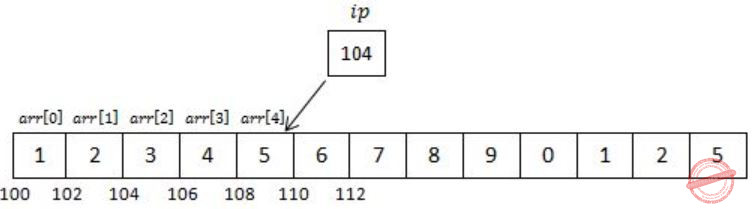We know that arr is a pointer to arr[ ] & hence arr+4 is pointer to 4th index of array (starting from 0 to 4).
Now *ip is a pointer of int type pointing to memory location 108, which is part of arr.
Hence, when we will print ip it will be equivalent to *(ip+1).
Address of ip will be incremented by 1 & value inside 110 will be printed.
 Question 17

A certain processor uses a fully associative cache of size 16 kB. The cache block size is 16 bytes. Assume that the main memory is byte addressable and uses a 32-bit address. How many bits are required for the Tag and the Index fields respectively in the addresses generated by the processor?

 A 28 bits and 0 bits B 24 bits and 4 bits C 24 bits and 0 bits D 28 bits and 4 bits
Computer-Organization       Cache
Question 17 Explanation:
Since, it is said for fully associative cache soNo index bits is there. So, now for tag bits,
Total bits - Offset bits = 32 - 4 = 28
So, tag bits = 28, Index bits = 0
 Question 18

Consider the following two statements about database transaction schedules:

I. Strict two-phase locking protocol generates conflict serializable schedules that are also recoverable.
II. Timestamp-ordering concurrency control protocol with Thomas Write Rule can generate view serializable schedules that are not conflict serializable.

Which of the above statements is/are TRUE?

 A Both I and II B Neither I nor II C II only D I only
Database-Management-System       Transactions
Question 18 Explanation:
(Memory-based question)
In strict 2PL, a transaction T does not release any of its exclusive (write) locks until after it commits or aborts.
Hence, no other transaction can read or write an item that is written by T unless T has committed, leading to a strict schedule for recoverability.
(Ref: Fundamentals of Database Systems by Elmasri and Navathe, 7e Pg. No. 789)
By ignoring the write, Thomas write rule allows schedules that are not conflict serializable but are nevertheless correct.
Those non-conflict-serializable schedules allowed satisfy the definition of view serializable schedules.
(Ref: Database System Concepts by Silberschatch, Korth and Sudarshan, 6e Pg No. 686)
 Question 19

Consider the grammar given below:

S → Aa
A → BD
B → b | ε
D → d | ε

Let a, b, d and \$ be indexed as follows:Compute the FOLLOW set of the non-terminal B and write the index values for the symbols in the FOLLOW set in the descending order. (For example, if the FOLLOW set is {a, b, d, \$}, then the answer should be 3210)

 A 30 B 31 C 10 D 21
Compiler-Design       Parsers
Question 19 Explanation:
Follow(B) = First(D) Union Follow(A)
{Follow(B) = Follow(A) when D is epsilon}
Follow(B) = {d} Union {a} = {a,d}
 Question 20

Let G be an arbitrary group. Consider the following relations on G:

R1: ∀a,b ∈ G, aR1b if and only if ∃g ∈ G such that a = g-1bg
R2: ∀a,b ∈ G, aR2b if and only if a = b-1

Which of the above is/are equivalence relation/relations?

 A R2 only B R1 and R2 C Neither R1 and R2 D R1 only
Engineering-Mathematics       Set-Theory
Question 20 Explanation:
A relation between the elements of a set is symmetric, reflexive and transitive then such relation is called as equivalence relation.
Consider Statement R1:
Reflexive:
aR1a
⇒ a = g-1ag
Left multiply both sides by g
⇒ ga = gg-1ag
Right multiply both sides by g-1
⇒ gag-1 = gg-1agg-1
⇒ gag-1 = a [∴ The relation is reflexive]
Symmetric:
If aR1b, then ∃g ∈ G such that gag-1 = b then a = g-1bg, which is Correct.
⇒ So, given relation is symmetric.
Transitive:
The given relation is Transitive.
So, the given relation R1 is equivalence.
R2:
The given relation is not reflexive.
So, which is not equivalence relation.
Such that a ≠ a-1.
So, only R1 is true.
 Question 21

In 16-bit 2's complement representation, the decimal number -28 is:

 A 1111 1111 1110 0100 B 1111 1111 0001 1100 C 0000 0000 1110 0100 D 1000 0000 1110 0100
Digital-Logic-Design       Number-Systems
Question 21 Explanation:
+28 = 0000 0000 0001 1100

1’s complement = 1111 1111 1110 0011
2’s complement = 1’s complement + 1

2’s complement = 1111 1111 1110 0100 = (-28)
 Question 22

Two numbers are chosen independently and uniformly at random from the set {1, 2, ..., 13}. The probability (rounded off to 3 decimal places) that their 4-bit (unsigned) binary representations have the same most significant bit is ______.

 A 0.502 B 0.461 C 0.402 D 0.561
Digital-Logic-Design       Number-Systems
Question 22 Explanation:
1 - 0001
2 - 0010
3 - 0011
4 - 0100
5 - 0101
6 - 0110
7 - 0111
8 - 1000
9 - 1001
10 - 1010
11 - 1011
12 - 1100
13 - 1101
The probability that their 4-bit binary representations have the same most significant bit is
= P(MSB is 0) + P(MSB is 1)
= (7×7)/(13×13) + (6×6)/(13×13)
= (49+36)/169
= 85/169
= 0.502
 Question 23

Which one of the following kinds of derivation is used by LR parsers?

 A Leftmost in reverse B Rightmost in reverse C Leftmost D Rightmost
Compiler-Design       Parsers
Question 23 Explanation:
LR parsers have Rightmost derivation in reverse.
 Question 24

Consider a sequence of 14 elements: A = [-5, -10, 6, 3, -1, -2, 13, 4, -9, -1, 4, 12, -3, 0]. The subsequence sum. Determine the maximum of S(i,j), where 0 ≤ i ≤ j < 14. (Divide and conquer approach may be used)

 A 19 B 39 C 29 D 09
Algorithms       Dynamic-Programming
Question 24 Explanation:
First understand the subsequence is an array is
Ex:
{A, B, C, D}
{A, AB, AC, AD, ABC, ABD, ACD, B, BC, BD, BCD, C, CD, D }
Step-1: Array of elements A = [-5, -10, 6, 3, -1, -2, 13, 4, -9, -1, 4, 12, -3, 0 ]
Step-2: As per the given question, if they want to find maximum subsequence means
{6,3,13,4,4,12}
= 42
Step-3: But according to given recurrence relation, the sequence should be continuous. {6,3,13,4,4,12}.
This is not continuous subsequence.
Step-4: The continuous sequence is {6, 3, -1, -2, 13, 4, -9, -1, 4, 12}
Total is {29}.
Note: We can't get more than 29 maximum subsequence sum.
 Question 25

Consider three concurrent processes P1, P2 and P3 as shown below, which access a shared variable D that has been initialized to 100.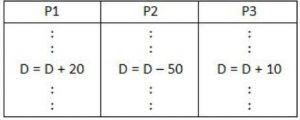The process are executed on a uniprocessor system running a time-shared operating system. If the minimum and maximum possible values of D after the three processes have completed execution are X and Y respectively, then the value of Y–X is _______.

 A 10 B 40 C 60 D 80
Operating-Systems       Process-Synchronization
Question 25 Explanation:P1 executes D=D+20, D=120.
P3 executes D=D+10, D=130.
Now, P2 has D=100, executes
D = D-50 = 100-50 = 50
P2 writes D=50 final value. This is minimum.
Next,
P2 reads D=100, executes D = D-50, before that assume P1 & P3 has read D=100.
P2 makes D=50 & writes it.
P1 executes (D=100), D=D+20 & P3 executes D=D+10 gives maximum value D=130.
So, Y - X = 130 - 50 =80.
 Question 26

An array of 25 distinct elements is to be sorted using quicksort. Assume that the pivot element is chosen uniformly at random. The probability that the pivot element gets placed in the worst possible location in the first round of partitioning (rounded off to 2 decimal places) is _____.

 A 0.08 B 0.01 C 1 D 8
Algorithms       Sorting
Question 26 Explanation:
Step-1: Given, 25 distinct elements are to be sorted using quicksort.
Step-2: Pivot element = uniformly random.
Step-3: Worst case position in the pivot element is either first (or) last.
Step-4: So total 2 possibilities among 25 distinct elements
= 2/25
= 0.08
 Question 27

Let X be a square matrix. Consider the following two statements on X.

I. X is invertible.
II. Determinant of X is non-zero.

Which one of the following is TRUE?

 A I implies II; II does not imply I. B II implies I; I does not imply II. C I and II are equivalent statements. D I does not imply II; II does not imply I.
Engineering-Mathematics       Linear-Algebra
Question 27 Explanation:
X is invertible means, that X is non-singular matrix.
That means we can also say that determinant of X is non-zero.
 Question 28

For Σ = {a,b}, let us consider the regular language L = {x|x = a2+3k or x = b10+12k, k ≥ 0}. Which one of the following can be a pumping length (the constant guaranteed by the pumping lemma) for L?

 A 3 B 9 C 5 D 24
Theory-of-Computation       Regular-Language
Question 28 Explanation:
Pumping Lemma for Regular Languages:
For any language L, there exists an integer n, such that for all x ∈ L with |x| ≥ n, there exists u,v, w ∈ Σ*, such that x = uvw, and
(1) |uv| ≤ n
(2) |v| ≥ 1
(3) for all i ≥ 0: uviw ∈ L
We have to find "n" which satisfies for all the strings in L.
Considering strings derived by b10+12k.
The minimum string in L = "bbbbbbbbbb" but this string b10 cannot be broken in uvw.
So, pumping length 3, 9 and 5 cannot be the correct answer.
So, the minimum pumping length, such that any string in L can be divided into three parts "uvw" must be greater than 10.
 Question 29

Let G be an undirected complete graph on n vertices, where n > 2. Then, the number of different Hamiltonian cycles in G is equal to

 A n! B 1 C (n-1)! D
Engineering-Mathematics       Graph-Theory
Question 29 Explanation:
A Hamiltonian cycle is a closed loop on a graph where every node (vertex) is visited exactly once.
The total number of hamiltonian cycles in a complete graph are
(n-1)!/2, where n is number of vertices.
 Question 30

Consider Z = X - Y, where X, Y and Z are all in sign-magnitude form. X and Y are each represented in n bits. To avoid overflow, the representation of Z would require a minimum of:

 A n bits B n + 2 bits C n - 1 bits D n + 1 bits
Digital-Logic-Design       Number-Systems
Question 30 Explanation:
In case of addition of two numbers with the same sign, there is a chance of overflow.
To store overflow/carry bit there should be extra space to accommodate it.
Hence, Z should be n+1 bits.
 Question 31

The value of 351 mod 5 is ______.

 A 3 B 5 C 2 D 1
Computer-Networks       Network-Security
Question 31 Explanation:
351 mod 5
⇒ 31 = 3 ⇒ 3 mod 5 = 3
32 ⇒ 9 mod 5 = 4
33 ⇒ 27 mod 5 = 2
34 ⇒ 81 mod 5 = 1
35 ⇒ 243 mod 5 = 3
For every four numbers sequence is repeating.
So, (51 % 4) = 3
⇒ 33 = 27
⇒ 27 mod 5 = 2
 Question 32

Which one of the following is NOT a valid identity?

 A (x + y) ⊕ z = x ⊕ (y + z) B (x ⊕ y) ⊕ z = x ⊕ (y ⊕ z) C x ⊕ y = x + y, if xy = 0 D x ⊕ y = (xy + x'y')'
Digital-Logic-Design       Boolean-Expression
Question 32 Explanation:
Let x=1, y=1, z=0.
(x+y) ⊕ z = (1+1)⊕ 0 = 1 ⊕ 0 = 1
x ⊕ (y+z) = 1⊕(1+0) = 1 ⊕ 1 = 0
So,
(x+y) ⊕ z ≠ x ⊕ (y+z)
 Question 33

Consider the following C program:

```         #include <stdio.h>
int jumble (int x, int y)  {
x = 2 * x + y ;
return x ;
}
int main ( )  {
int x=2, y=5 ;
y = jumble (y, x) ;
x = jumble (y, x) ;
printf ("%d \n", x) ;
return 0 ;
}
```

The value printed by the program is ______.

 A 26 B 67 C 25 D 13
Programming-for-Output-Problems       Functions
Question 33 Explanation:
////////////////////////////////////Program
#include
int jumble(int x, int y)
{
printf("Inside jumble : 2*%d + %d\n", x,y);
x = 2*x +y;
return x;
}
int main()
{
int x=2, y=5;
printf("Initial x=%d, y=%d\n",x,y);
printf("1st jumble call : jumble(%d,%d)\n",y,x);
y = jumble(y,x);
printf("Value of y after 1st jumble = %d\n", y);
printf("2nd jumble call: jumble(%d,%d)\n", y,x);
x = jumble(y,x);
printf("Value of x after 2nd jumble = %d\n", x);
printf("Final : %d\n", x);
return 0;
}
////////////////////////////////////OUTPUT
Initial x=2, y=5
1st jumble call: jumble(5,2)
Inside jumble : 2*5 + 2
Value of y after 1st jumble = 12
2nd jumble call: jumble(12,2)
Inside jumble : 2*12 + 2
Value of x after 2nd jumble = 26
Final : 26
 Question 34

The following C program is executed on a Unix/Linux system:

```            #include <unistd.h>
int main ()
{
int i ;
for (i=0; i<10; i++)
if (i%2 == 0) fork ( ) ;
return 0 ;
}
```

The total number of child processes created is _____.

 A 26 B 33 C 31 D 28
Operating-Systems       System-Calls
Question 34 Explanation:
Fork( ) statement is executed when 'i' is even. Thus the above code is equivalent to
#include
int main( )
{
int i;
fork( );
fork( );
fork( );
fork( );
fork( );
}
n - fork statements will have 2n-1 child.
Hence, n = 5 ⇒ We have 31 child processes.
 Question 35

Let U = {1,2,...,n}. Let A = {(x,X)|x ∈ X, X ⊆ U}. Consider the following two statements on |A|.Which of the above statements is/are TRUE?

 A Only II B Only I C Neither I nor II D Both I and II
Engineering-Mathematics       Set-Theory
Question 35 Explanation:
Let us consider U = {1, 2}
and given A = {(x, X), x∈X and X⊆U}
Possible sets for U = {Φ, {1}, {2}, {1, 2}}
if x=1 then no. of possible sets = 2
x=2 then no. of possible sets = 2
⇒ No. of possible sets for A = (no. of sets at x=1) + (no. of sets at x=2) = 2 + 2 = 4
Consider statement (i) & (ii) and put n=2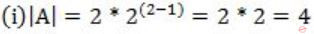Statement (i) is true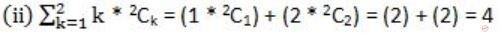Statement (i) and (ii) both are true.
 Question 36

Consider the following relations P(X,Y,Z), Q(X,Y,T) and R(Y,V).How many tuples will be returned by the following relational algebra query?

```          ∏x(σ(P.Y=R.Y ∧ R.V=V2)(P × R)) - ∏x(σ(Q.Y=R.Y ∧ Q.T>2)(Q × R))
```
 A 0 B 1 C 2 D 3
Database-Management-System       Relational-Algebra
Question 36 Explanation:
σ(P.Y = R.Y ∧ R.V = V2)(P × R)x(P.Y = R.Y ∧ R.V = V2)(P × R))σ(Q.Y = R.Y ∧ Q.T>2)(Q × R)x(Q.Y = R.Y ∧ Q.T>2)(Q × R))x(P.Y = R.Y ∧ R.V = V2)(P × R)) - ∏x(Q.Y = R.Y ∧ Q.T>2)(Q × R))Question 37

What is the minimum number of 2-input NOR gates required to implement a 4-variable function function expressed in sum-of-minterms form as f = Σ(0, 2, 5, 7, 8, 10, 13, 15)? Assume that all the inputs and their complements are available.

 A 2 B 4 C 7 D 1 E 3(Option not given)
Digital-Logic-Design       Logic-Gates
Question 37 Explanation:
f = Σ(0, 2, 5, 7, 8, 10, 13, 15)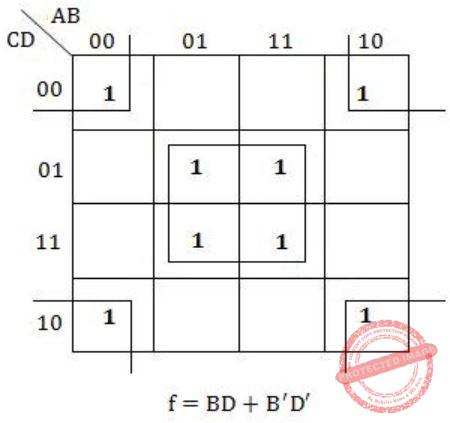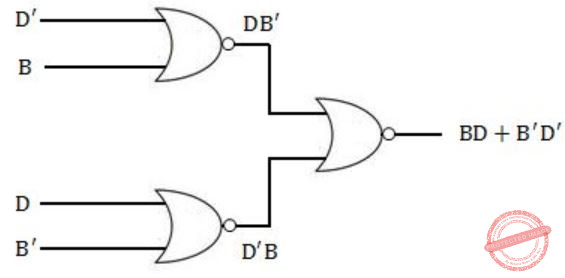Question 38

Consider three machines M, N and P with IP addresses 100.10.5.2, 100.10.5.5, and 100.10.5.6 respectively. The subnet mask is set to 255.255.255.252 for all the three machines. Which one of the following is true?

 A M, N, and P all belong to the same subnet B Only M and N belong to the same subnet C M, N and P belong to three different subnets D Only N and P belong to the same subnet
Question 38 Explanation:
Take each IP and do bitwise AND with the given Subnet Mask. If we get the same network ID for the given IP'S then it will belong to the same subnet.Therefore, N and P belong to the same subnet.
 Question 39

Consider three 4-variable functions f1, f2 and f3, which are expressed in sum-of-minterms as

```f1 = Σ(0, 2, 5, 8, 14),  f2 = Σ(2, 3, 6, 8, 14, 15),  f3 = Σ(2, 7, 11, 14)
```

For the following circuit with one AND gate and one XOR gate, the output function f can be expressed as: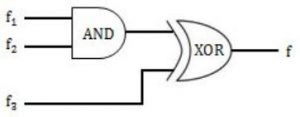A Σ (2, 14) B Σ (7, 8, 11) C Σ (2, 7, 8, 11, 14) D Σ (0, 2, 3, 5, 6, 7, 8, 11, 14, 15)
Digital-Logic-Design       Logic-Gates
Question 39 Explanation:
f1*f2 = ∑(2,8,14)
f3 = ∑(2,7,11,14)
f1*f2 ⊕ f3 = ∑(2,8,14) ⊕ ∑(2,7,11,14)
= ∑(8,7,11)
(Note: Choose the terms which are not common)
 Question 40

Consider the augmented grammar given below:

```    S' → S
S → 〈L〉 | id
L → L,S | S
```

Let I0 = CLOSURE ({[S' → ·S]}). The number of items in the set GOTO (I0 , 〈 ) is: _____.

 A 4 B 5 C 6 D 7
Compiler-Design       Parsers
Question 40 Explanation:
I0 = CLOSURE ({[S' → ·S]})Hence, the set GOTO (I0 , 〈 ) has 5 items.
 Question 41

Consider the following statements:

I. The smallest element in a max-heap is always at a leaf node.
II. The second largest element in a max-heap is always a child of the root node.
III. A max-heap can be constructed from a binary search tree in Θ(n) time.
IV. A binary search tree can be constructed from a max-heap in Θ(n) time.

Which of the above statements are TRUE?

 A I, II and III B II, III and IV C I, III and IV D I, II and IV
Data-Structures       Binary-Trees
Question 41 Explanation:
i) TRUE: The smallest element in heap is always a leaf node but depends upon the graph, it may be left or right side of the graph.
(ii) TRUE: The second smallest element in a heap is always a child of root node.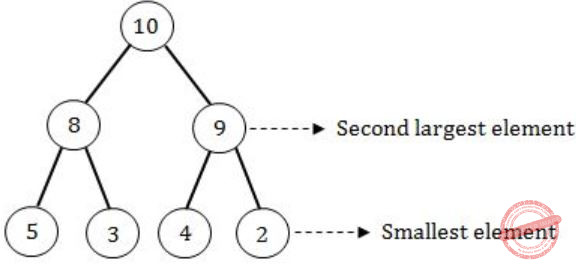(iii) TRUE: Converting from binary search tree to max heap will take O(n) time as well as O(n) space complexity.
(iv) FALSE: We can’t convert max heap to binary search tree in O(n) time.
 Question 42

In an RSA cryptosystem, the value of the public modulus parameter n is 3007. If it is also known that Φ(n) = 2880, where Φ() denotes Euler's Quotient Function, then the prime factor of n which is greater than 50 is ______.

 A 107 B 97 C 45 D 92
Computer-Networks       Network-Security
Question 42 Explanation:
It can be solved by Hit and trial method in less time.
n = 3007, fi(n) = 2880 → fi(n) = (p – 1) (q – 1),
where p, q are prime factor of n.
The unit place of n is 7, it is a prime number and factor will be
1.7=7
11*17
21*37
31*47
….
31*97 =>3007
n = 3007 => 31*97
Therefore, 31 & 97 are the two prime numbers, which is satisfying the condition and 97 is greater than 50.
So, 97 is the correct answer.
Other methods:
When ϕ(n) is given when n=pq where p and q are prime numbers, then we have
ϕ(n) = (p−1)(q−1) = pq−(p+q)+1
But pq=n,
therefore, ϕ(n) = n−(p+q)+1 and p+q = n+1−ϕ(n).
Now, p and q are the roots of the equation,
x2 − (p+q)x + pq = (x-p)(x-q)
Substituting for p+q and pq in the above equation
x2 - (n+1-ϕ(n))x + n
 Question 43

A relational database contains two tables Student and Performance as shown below:The primary key of the Student table is Roll_no. For the Performance table, the columns Roll_no. and Subject_code together from the primary key. Consider the SQL query given below:

```SELECT S.Student_name, sum (P.Marks)
FROM Student S, Performance P
WHERE P.Marks > 84
GROUP BY S.Student_name;```

The number of rows returned by the above SQL query is _____.

 A 0 B 9 C 7 D 5
Database-Management-System       SQL
Question 43 Explanation:
(Executed under Oracle Express Edition)
SQL> SELECT S.Student_name,sum(P.Marks)
2 FROM Student S,Performance P
3 WHERE P.Marks>84
4 GROUP BY S.Student_name;Question 44

Assume that in a certain computer, the virtual addresses are 64 bits long and the physical addresses are 48 bits long. The memory is word addressable. The page size is 8 kB and the word size is 4 bytes. The Translation Look-aside Buffer (TLB) in the address translation path has 128 valid entries. At most how many distinct virtual addresses can be translated without any TLB miss?

 A 8×220 B 4×220 C 16×210 D 256×210
Operating-Systems       Memory-Management
Question 44 Explanation:
A TLB has 128 valid entries.
So, it can refer to 27 pages.
Each page size is 8 kB & word is 4 bytes.Question 45

Consider the following four processes with arrival times (in milliseconds) and their length of CPU bursts (in milliseconds) as shown below: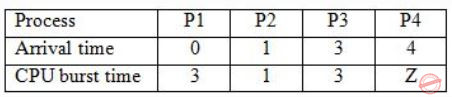These processes are run on a single processor using preemptive Shortest Remaining Time First scheduling algorithm. If the average waiting time of the processes is 1 millisecond, then the value of Z is _____.

 A 2 B 7 C 1 D 4
Operating-Systems       Process-Scheduling
Question 45 Explanation:
This is the Gantt chart till time = 4 unitsAt this point P4 arrives with burst 'Z' & P3 is in queue with burst 3.
P1 & P2 have executed with P1 incurred delay 1unit & P2 0units.
Hence, Avg = 0+1+x/4 =1
⇒ x=3, the next delay should be 3. It would happen if assume Z=2.
It executes and completes at 6.
P3 will wait totally for 3units.
Hence, Avg=1.Z=2
 Question 46

Let T be a full binary tree with 8 leaves. (A full binary tree has every level full). Suppose two leaves a and b of T are chosen uniformly and independently at random. The expected value of the distance between a and b in T (i.e., the number of edges in the unique path between a and b) is (rounded off to 2 decimal places) _____.

 A 5.54 B 1.34 C 4.25 D 3.82
Data-Structures       Binary-Trees
Question 46 Explanation:
There can be 8 paths between any 2 uniformly & independently chosen leaf nodes.
A node can be chosen twice and the path from that node to itself will be zero.
∴ Path 1 = 0
Similarly,
Path 2 = 2
Path 3 = 4
Path 4 = 4
Path 5 = 6
Path 6 = 6
Path 7 = 6
Path 8 = 6
∴ Expected value = Σ Path length × Probability of selecting path
= 2×1/8 + 4×2/8 + 6×4/8 + 0×1/8
= 1/4 + 1/1 + 3/1 + 0
= 4 + 1/4
= 17/4
= 4.25
 Question 47

Consider that 15 machines need to be connected in a LAN using 8-port Ethernet switches. Assume that these switches do not have any separate uplink ports. The minimum number of switches needed is _____.

 A 3 B 7 C 1 D 5
Computer-Networks       Ethernet
Question 47 Explanation:
In 8 port Ethernet switch one port for the network connection and remaining 7 port for the machine.
Therefore, the total required number of the switches = Ceil (15 /7) = 3Question 48

There are n unsorted arrays: A1, A2, ..., An. Assume that n is odd. Each of A1, A2, ..., An contains n distinct elements. There are no common elements between any two arrays. The worst-case time complexity of computing the median of the medians of A1, A2, ..., An is

 A O(n) B O(n log n) C Ω(n2 log n) D O(n2)
Algorithms       Sorting
Question 48 Explanation:
Finding the median in an unsorted array is O(n).
But it is similar to quicksort but in quicksort, partitioning will take extra time.
→ Find the median will be (i+j)/2
1. If n is odd, the value is Ceil((i+j)/2)
2. If n is even, the value is floor((i+j)/2)
-> Here, total number of arrays are
⇒ O(n)*O(n)
⇒ O(n2)
Note:
They are clearly saying that all are distinct elements.
There is no common elements between any two arrays.
 Question 49

Suppose Y is distributed uniformly in the open interval (1,6). The probability that the polynomial 3x2 + 6xY + 3Y + 6 has only real roots is (rounded off to 1 decimal place) _____.

 A 0.3 B 0.9 C 0.1 D 0.8
Engineering-Mathematics       Probability
Question 49 Explanation:
Given polynomial equation is
3x2 + 6xY + 3Y + 6
= 3x2 + (6Y)x + (3Y + 6)
which is in the form: ax2 + bx + c
For real roots: b2 - 4ac ≥ 0
⇒ (6Y)2 - 4(3)(3Y + 6) ≥ 0
⇒ 36Y2 - 36Y - 72 ≥ 0
⇒ Y2 - Y - 2 ≥ 0
⇒ (Y+1)(Y-2) ≥ 0
Y = -1 (or) 2
The given interval is (1,6).
So, we need to consider the range (2,6).
The probability = (1/(6-1)) * (6-2) = 1/5 * 4 = 0.8
 Question 50

Consider the following C program:

```       #include <stdio.h>
int main()
{
int a[] = {2, 4, 6, 8, 10} ;
int i, sum = 0, *b = a + 4 ;
for (i = 0; i < 5; i++)
sum = sum + (*b - i) - *(b - i) ;
printf ("%d\n", sum) ;
return 0 ;
}
```

The output of the above C program is _____.

 A 3 B 7 C 11 D 10
Programming-for-Output-Problems       Control-Statement
Question 50 Explanation:
///////////////////////////////////PROGRAM
#include
int main()
{
int a[] = {2,4,6,8,10};
int i, sum = 0, *b = a+4;
for(i=0; i<5; i++)
{ printf("*b, (*b-i): %d , %d\n",*b, (*b-i) );
printf("*(b-i): %d\n",*(b-i) );
printf("sum = %d + %d - %d\n",sum, (*b-i),*(b-i));
sum = sum + (*b-i) - *(b-i);
printf("sum = %d\n", sum);
}
printf("%d\n", sum);
return 0;
}
//////////////////////////////OUTPUT
*b, (*b-i): 10 , 10
*(b-i): 10
sum = 0 + 10 - 10
sum = 0
*b, (*b-i): 10 , 9
*(b-i): 8
sum = 0 + 9 - 8
sum = 1
*b, (*b-i): 10 , 8
*(b-i): 6
sum = 1 + 8 - 6
sum = 3
*b, (*b-i): 10 , 7
*(b-i): 4
sum = 3 + 7 - 4
sum = 6
*b, (*b-i): 10 , 6
*(b-i): 2
sum = 6 + 6 - 2
sum = 10
10
 Question 51

Let the set of functional dependencies F = {QR → S, R → P, S → Q} hold on a relation schema X = (PQRS). X is not in BCNF. Suppose X is decomposed into two schemas Y and Z, where Y = (PR) and Z = (QRS).

Consider the two statements given below.

I. Both Y and Z are in BCNF
II. Decomposition of X into Y and Z is dependency preserving and lossless

Which of the above statements is/are correct?

 A I only B Neither I nor II C II only D Both I and II
Database-Management-System       Normalization
Question 51 Explanation:
Y = (PR)
R → P
R+ = RP
* In R → P, 'R' is a super key. So, Y is in BCNF.
Z = (QRS)
QR → S
S → Q
CK's = QR, RS
* In, S → Q, 'S' is not a super key. So, Z is not in BCNF.
* Y is in BCNF and Z is not in BCNF.
* 'R' is common attribute in the relations Y and Z. and R is candidate key for Y. So, the decomposition is lossless.
* The FD, R → P is applicable on Y and QR → S, S → Q are applicablein 2.
So, the decomposition is dependency preserving.
* Hence, Statement II is correct.
 Question 52

Consider the first order predicate formula φ:

∀x[(∀z z|x ⇒ ((z = x) ∨ (z = 1))) ⇒ ∃w (w > x) ∧ (∀z z|w ⇒ ((w = z) ∨ (z = 1)))]

Here 'a|b' denotes that 'a divides b', where a and b are integers. Consider the following sets:

S1.  {1, 2, 3, ..., 100}
S2.  Set of all positive integers
S3. Set of all integers

Which of the above sets satisfy φ?

 A S1 and S3 B S1, S2 and S3 C S2 and S3 D S1 and S2
Engineering-Mathematics       Propositional-Logic
Question 52 Explanation:
The first order logic gives the meaning that if z is a prime number then there exists another prime number in the set which is larger than it.
One of the case:
If -7 is a number which is prime (either divided by -7 or 1 only). then there exists some number like -3 which is larger than -7 also satisfy the property (either divided by -3 or 1 only).
So, S3 is correct
It's true for all integers too.
 Question 53

A certain processor deploys a single-level cache. The cache block size is 8 words and the word size is 4 bytes. The memory system uses a 60-MHz clock. To service a cache miss, the memory controller first takes 1 cycle to accept the starting address of the block, it then takes 3 cycles to fetch all the eight words of the block, and finally transmits the words of the requested block at the rate of 1 word per cycle. The maximum bandwidth for the memory system when the program running on the processor issues a series of read operations is ______ × 106 bytes/sec.

 A 160 B 145 C 172 D 124
Computer-Organization       Cache
Question 53 Explanation:Cache block = 8 words
Word size = 4 bytes
Cache block size = 32 bytes
Clock = 60 MHz
⇒ T = 1/clock = 1/60×106 seconds
Cache miss
= 1 cycle(Address) + 3 cycles (8 words) + 1word/cycle ×8 (transfer)
= 12 cycles
= 12/60×106
Total bandwidth = total data/total time = 32 bytes/(12/60×106) = 160 × 106 bytes/second
 Question 54

Consider the following sets:

S1.  Set of all recursively enumerable languages over the alphabet {0,1}
S2.  Set of all syntactically valid C programs
S3.  Set of all languages over the alphabet {0,1}
S4.  Set of all non-regular languages over the alphabet {0,1}

Which of the above sets are uncountable?

 A S2 and S3 B S3 and S4 C S1 and S4 D S1 and S2
Theory-of-Computation       Countability
Question 54 Explanation:
S1 is countable, set of all recursively enumerable languages means set of all Turing machines and we can enumerate TM and have one to one correspondence between natural number.
S2 is countable, since a valid C program represents a valid algorithm and every algorithm corresponds to a Turing Machine, so S2 is equivalent to set of all Turing Machines.
S3 is is uncountable, it is proved by diagonalization method.
S4 is uncountable, as set of non-regular languages will have languages which is set of all languages over alphabet {0,1} i.e., S3.
 Question 55

Consider the following grammar and the semantic actions to support the inheritance type declaration attributes. Let X1, X2, X3, X4, X5 and X6 be the placeholders for the non-terminals D, T, L or L1 in the following table:Which one of the following are the appropriate choices for X1, X2, X3 and X4?

 A X1 = L, X2 = L, X3 = L1, X4 = T B X1 = L, X2 = T, X3 = L1, X4 = L C X1 = T, X2 = L, X3 = L1, X4 = T D X1 = T, X2 = L, X3 = T, X4 = L1
Compiler-Design       Synthesized-and-L-Attribute
Question 55 Explanation:
Since the production,
L → L1, id {X3.type = X4.type } , this production has L and L1, hence X3 and X4 cannot be T.
So option 1, 3 and 4 cannot be correct.
 Question 56

Which one of the following languages over Σ = {a,b} is NOT context-free?

 A {wwR |w ∈ {a,b}*} B {wanwRbn |w ∈ {a,b}*, n ≥ 0} C {anbi | i ∈ {n, 3n, 5n}, n ≥ 0} D {wanbnwR |w ∈ {a,b}*, n ≥ 0}
Theory-of-Computation       Context-Free-Language
Question 56 Explanation:
{wanwRbn |w ∈ {a,b}*, n ≥ 0} cannot be CFL.
This is similar to language
L = {anbmcndm | n, m > 0}
Suppose we push “w” then an and then wR, now we cannot match bn with an, because in top of stack we have wR.
 Question 57

Consider the following C program:

```             #include <stdio.h>
int r()  {
static int num=7 ;
return num-- ;
}
int main ()  {
for (r(); r (); r())
printf ("%d", r());
return 0 ;
}
```

Which one of the following values will be displayed on execution of the programs?

 A 41 B 63 C 52 D 630
Programming-for-Output-Problems       Control-Statement
Question 57 Explanation:
///////////////////////////PROGRAM
#include
int r()
{
int x;
static int num=7;
x =num--;
printf("num--: %d\n",x);
return x;
}
int main()
{
for(r(); r(); r())
{
printf("%d\n", r());
}
return 0;
}
//////////////////////////////OUTPUT
num--: 7
num--: 6
num--: 5
5
num--: 4
num--: 3
num--: 2
2
num--: 1
num--: 0
 Question 58

Suppose that in an IP-over-Ethernet network, a machine X wishes to find the MAC address of another machine Y in its subnet. Which one of the following techniques can be used for this?

 A X sends an ARP request packet to the local gateway's IP address which then finds the MAC address of Y and sends to X B X sends an ARP request packet with broadcast IP address in its local subnet C X sends an ARP request packet to the local gateway's MAC address which then finds the MAC address of Y and sends to X D X sends an ARP request packet with broadcast MAC address in its local subnet
Computer-Networks       ARP-RARP
Question 58 Explanation:
Address Resolution Protocol (ARP) is a protocol for mapping an Internet Protocol address (IP address) to a physical machine address (MAC) that is recognized in the local network.
Since both are present in the same subnet thus an ARP request packet can be sent as broadcast MAC address, all will see but the only destination will reply as a unicast reply.
Video Reference :
http://eclassesbyravindra.com/mod/page/view.php?id=147
 Question 59

Consider the following matrix: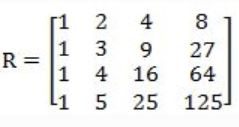The absolute value of the product of Eigen values of R is ______.

 A 12 B 17 C 10 D 8
Engineering-Mathematics       Linear-Algebra
Question 59 Explanation:Question 60

Consider the following C function.

```               void convert (int n) {
if (n < 0)
printf ("%d", n);
else {
convert (n/2);
printf ("%d", n%2);
}
}
```

Which one of the following will happen when the function convert is called with any positive integer n as argument?

 A It will print the binary representation of n in the reverse order and terminate. B It will not print anything and will not terminate. C It will print the binary representation of n and terminate. D It will print the binary representation of n but will not terminate.
Programming-for-Output-Problems       Functions
Question 60 Explanation:
////////////////OUTPUT
Sequence of function calls
Convert(6)
Convert(3)
Convert(1)
Convert(0)
:
Convert(0)
:
:
It will not terminate and never produce any output.
Note:
There is no instruction which stops the loop.
 Question 61

The index node (inode) of a Unix-like file system has 12 direct, one single-indirect and one double-indirect pointers. The disk block size is 4 kB, and the disk block address is 32-bits long. The maximum possible file size is (rounded off to 1 decimal place) ______ GB.

 A 7 B 9 C 2 D 4
Operating-Systems       File system-I/O-protection
Question 61 Explanation:
No. of Disk block pointers = 4kB/32bits = 1k
Max. file size
= (12 × 1k + 1k × 1k) × 4kB
≈ (1024 × 12 + 1024 × 1024) × 4 × 1024 bytes
≈ 4GB
 Question 62

Consider the following snapshot of a system running n concurrent processes. Process i is holding Xi instances of a resource R, 1 ≤ i ≤ n. Assume that all instances of R are currently in use. Further, for all i, process i can place a request for at most Yi additional instances of R while holding the Xi instances it already has. Of the n processes, there are exactly two processes p and q such that Yp = Yq = 0. Which one of the following conditions guarantees that no other process apart from p and q can complete execution?

 A Min (Xp, Xq) ≥ Min {Yk | 1 ≤ k ≤ n, k ≠ p, k ≠ q} B Xp + Xq < Max {Yk | 1 ≤ k ≤ n, k ≠ p, k ≠ q} C Min (Xp, Xq) ≤ Max {Yk | 1 ≤ k ≤ n, k ≠ p, k ≠ q} D Xp + Xq < Min {Yk | 1 ≤ k ≤ n, k ≠ p, k ≠ q}
Question 62 Explanation:
{P1, P2, ..., Pn}
Pi holds Xi instances.
Pi can request additional Yi instances.
Given two process p & q such that their additional requests are zero.
Yp = Yq = 0
{Yk | 1 ≤ k ≤ n, k ≠ p, k ≠ q} means that out of 'n' processes, we are left with (n-2) process (except p&q), i.e., Yk indicates additional request of all the processes (n-2) except p & q.
For p & q to complete first, accordingly
Xp + Xq < Min {Yk}
Option D is correct.
There are exactly two process p and q which do not need any additional instances of resources.
So, p and q will complete their execution and will release Xp and Xq instances of resources.
Now to guarantee that no other process apart from p and q can complete execution, the no. of instances of resources available must be less than the minimum no. of instances of resources required by any other process, i.e.,
Xp + Xq < Min {Yk | 1 ≤ k ≤ n, k ≠ p, k ≠ q}.
 Question 63

Let Σ be the set of all bijections from {1, ..., 5} to {1, ..., 5}, where id denotes the identity function i.e. id(j) = j,∀j. Let º denote composition on functions. For a string x = x1 x2 ... xn ∈ Σn, n ≥ 0. Let π(x) = x1 º x2 º ... º xn.

Consider the language L = {x ∈ Σ* | π(x) = id}. The minimum number of  states in any DFA accepting L is ______.

 A 120 B 136 C 125 D 132
Theory-of-Computation       Finite-Automata
 Question 64

Consider the following C program:

```       #include <stdio.h>
int main ()  {
float sum = 0.0, j = 1.0, i = 2.0;
while (i/j > 0.0625) {
j = j + j;
sum = sum + i/j;
printf ("%f \n", sum);
}
return 0;
}
```

The number of times the variable sum will be printed, when the above program is executed, is ______.

 A 5 B 2 C 7 D 10
Programming-for-Output-Problems       Control-Statement
Question 64 Explanation:
///////////////////////////////// PROGRAM
#include
int main()
{
float sum= 0.0, j=1.0, i=2.0;
while(i/j > 0.0625)
{
j = j+j;
sum = sum+i/j;
printf("%f\n",sum);
}
return 0;
}
//////////////////////////////////OUTPUT
1.000000
1.500000
1.750000
1.875000
1.937500
 Question 65

Let G be any connected, weighted, undirected graph.

I. G  has a unique minimum spanning tree, if no two edges of G have the same weight.
II. G  has a unique minimum spanning tree, if, for every cut of G, there is a unique minimum-weight edge crossing the cut.

Which of the above statements is/are TRUE?

 A I only B II only C Both I and II D Neither I nor II
Al
Question 65 Explanation:
Given G be a connected, weighted and undirected graph,
I. TRUE: G Graph is unique, no two edges of the graph is same.Step-1: Using Kruskal's algorithm, arrange each weights in ascending order.
17, 18, 20, 21, 22, 23, 24, 25, 26, 27, 28, 29, 30
Step-2: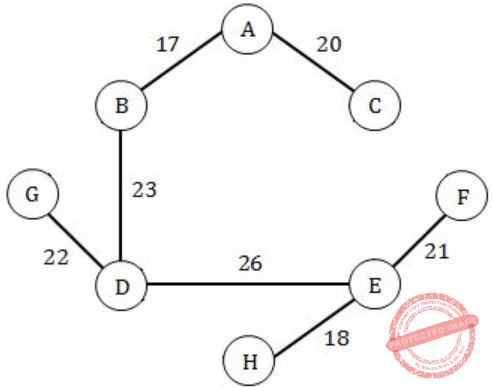Step-3: 17 + 18 + 20 + 21 + 22 + 23 + 26 = 147
Step-4: Here, all the elements are distinct. So, the possible MCST is 1.
II. TRUE: As per the above graph, if we are cut the edge, that should the be the minimum edge.
Because we are already given, all minimum edge weights if graph is distinct.
There are 65 questions to complete.